# 14.7.1 Astrometry

When we use clusters located farther than 1000 pc, we derive an average zero point difference with MSWC $(\varpi_{\rm Gaia}-\varpi_{\rm reference})=-0.059,-0.091$ mas for MWSC and DAML respectively, but with a large $\sigma\sim 0.2$. However, looking at the trends with colour and magnitude, we find a complex pattern. This is also evident looking at individual clusters, whose members are selected on the basis of the proper motions (see for instance Figure 14.70).

Looking at differential parallax to the cluster median $\Delta_{\rm par}$, for the whole sample of clusters located farther than 1000 pc, we get indication about zero point changes. When plotted versus $G$ jumps in the zero point at $G$ =11.5,13,14 are evident. Strong variations are evident for stars bluer and redder than $G_{\mathrm{BP}}-G_{\mathrm{RP}}$ $\sim 0.8$. When divided by the nominal uncertainty, these patterns are still present, with reduced amplitude, implying that nominal uncertainties on the parallax do not account for the zero point variation, i.e. nominal uncertainties are underestimated. More detail can be found in Fabricius et al. (2021).

Finally, we compare the parallaxes of single stars in Gaia DR2 and Gaia DR3. The median difference is ($\varpi_{\rm Gaia~{}DR3}-\varpi_{\rm Gaia~{}DR2})=0.017$ mas, ( with $16\%=-0.047$ mas; $84\%=0.082$ mas) on the whole sample, with a dependence on the magnitude.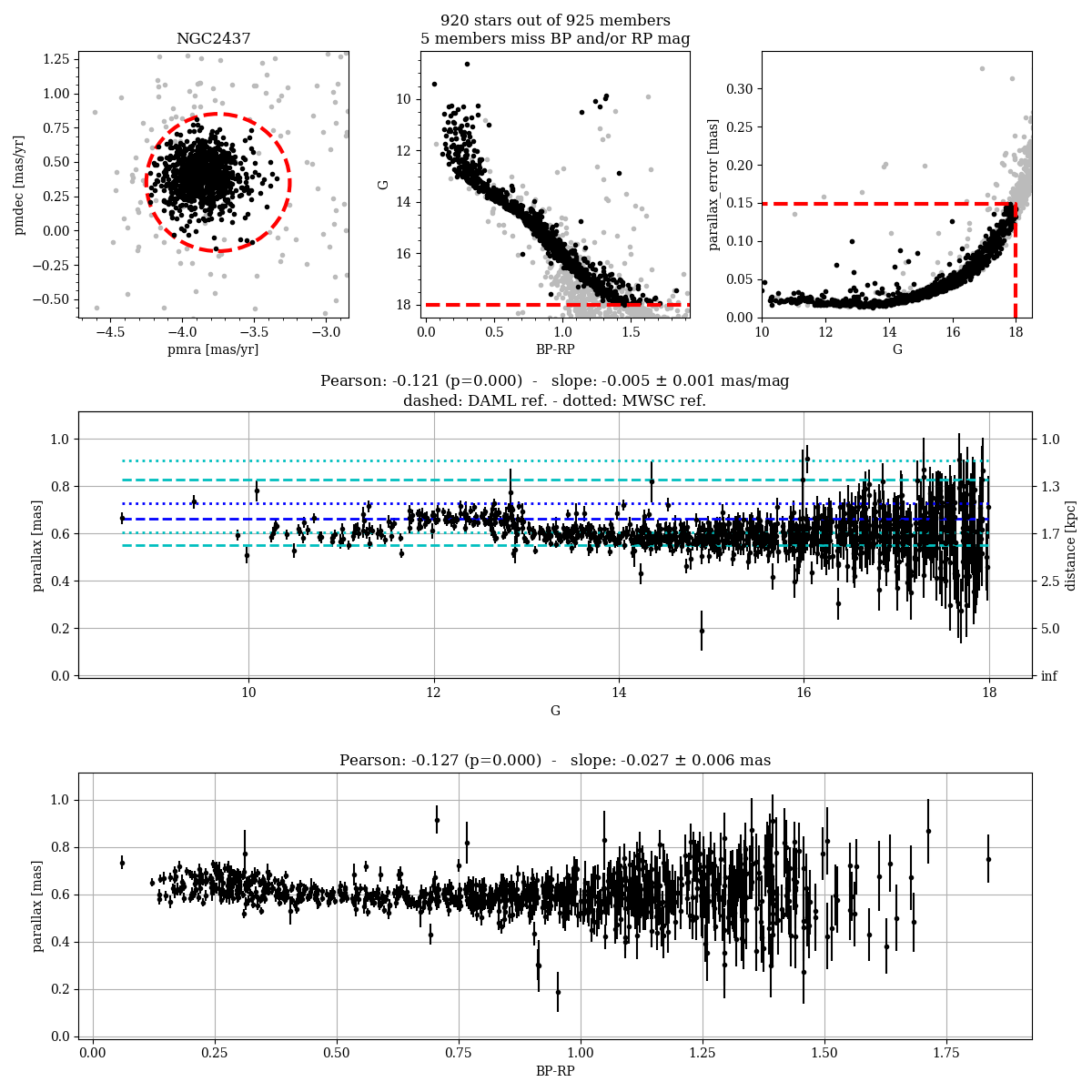Figure 14.70: Parallax trends for NGC2437 as a function of magnitude and colour.

## Astrometric precision of parallaxes

In Figure 14.71 we analysed the uncertainties on the parallax for clusters more distant than 1000 pc. We analyse the difference between Gaia DR3 parallaxes for single stars and the median parallax of the cluster normalised to the Gaia DR3 nominal error. The sigma of the distribution is larger than 1 ($\sim 1.2$) i.e. the uncertainties are underestimated. In particular, formal uncertainties underestimate the errors mainly for stars brighter than $G=14$. The distribution shows a bump at $G$ $\sim 13$.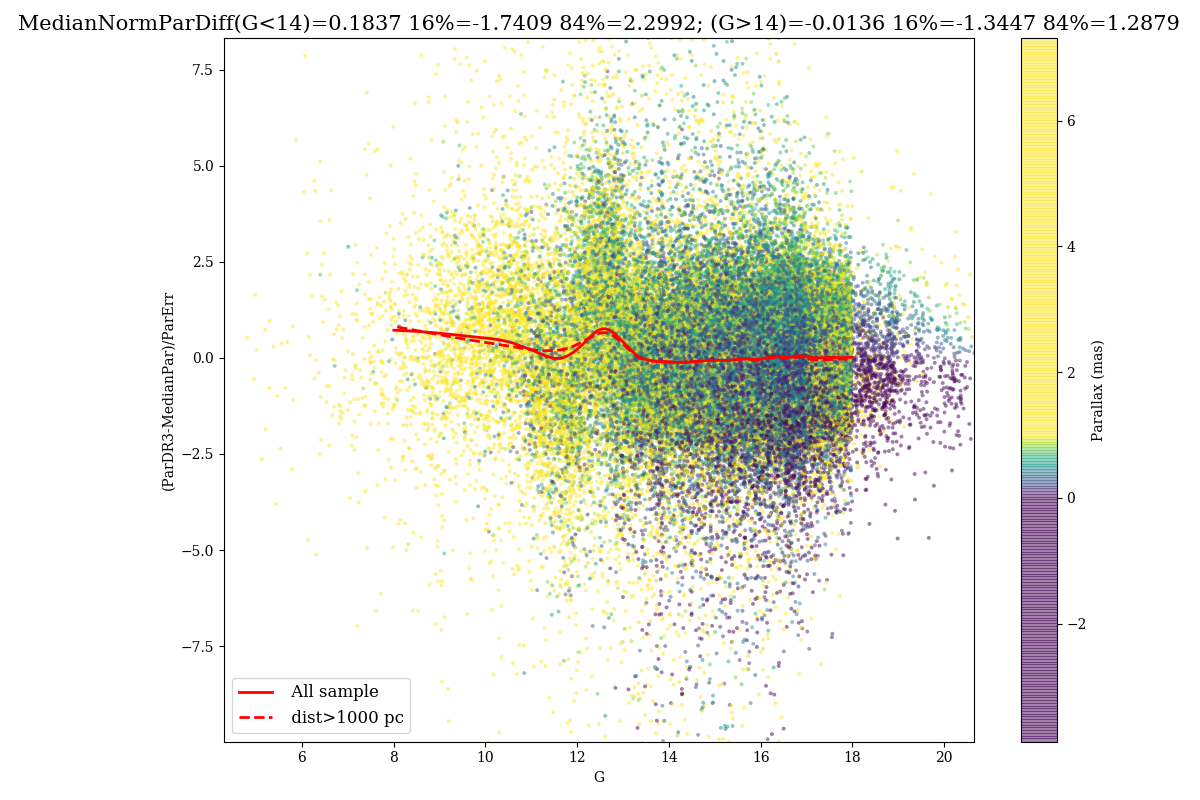Figure 14.71: Difference between Gaia DR3 parallaxes for single stars and the median parallax of the cluster normalised the Gaia DR3 nominal error vs G for the whole sample of stars in clusters. The red line is the LOWESS.

## Proper motions

Figure 14.72 shows the case of NGC 7099 compared with literature values. The zero point of proper motions presents a complex dependence on magnitude and colour. Similar patterns are evident for all the clusters. The dispersion of the proper motions is not accounted by the nominal uncertainties, i.e. nominal uncertainties are underestimated, mainly at the bright end ($G$ $<14$).

We calculate the proper motion differences (median) between Gaia DR3 and Gaia DR2 for the best cluster sample. The zero point median difference is $-0.077\pm 0.21$ $\rm\,mas\,yr^{-1}$ in right ascension and in declination on the whole sample.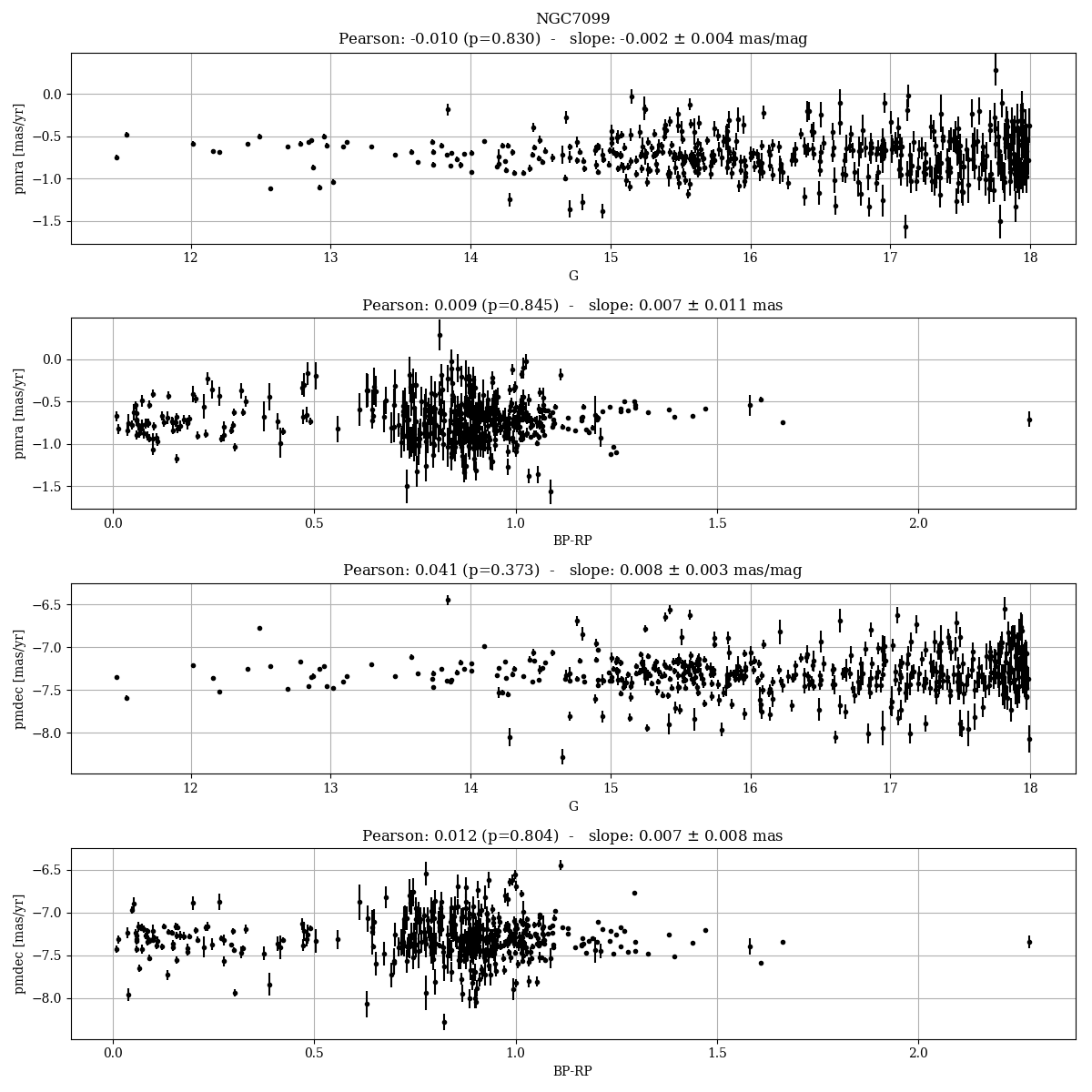Figure 14.72: Proper motion distribution in magnitude and colour for the cluster NGC 7099.

## Proper motion precision in crowded areas

We compare the proper motions in the centre of M4 with external HST data (Nascimbeni et al. 2014) , where high quality relative proper motions are available. The precision of HST proper motions is of the order of 0.33 $\rm\,mas\,yr^{-1}$. M4 stars are affected by crowding already at bright magnitudes ($G$ $>$ 16-17) (see Figure 14.73) In Figure 14.74 we present the scaled dispersion of the difference between Gaia and HST proper motions for the stars having different Gaia nominal uncertainties, and a moderate (c=2) to high ($c>5$) colour excess. The colour excess is taken as a proxy for the level of contamination. The scaled dispersion is very close to 1 for both $\mu_{\alpha}$ and $\mu_{\delta}$ for bright stars with little contamination, but it gets to 1.6-1.8 for faint stars with a high contamination level. This indicated that the nominal uncertainties are correctly estimated only for bright stars.Figure 14.73: Colour magnitude diagram of M4 from Gaia data. The colour indicates the colour excess.Figure 14.74: The scaled dispersion of the pms in M4 for the stars having increasing nominal uncertainties and colour excess.

## Integrated Photometry

CMD features

In Figure 14.75 we show the CMD of NGC 2516 where stars are selected on the basis of the pms. A hook is visible from $G$ $\sim 19$ and $G_{\mathrm{BP}}-G_{\mathrm{RP}}\sim 3$ where a number of stars have a too blue colour for their location in the main sequence. This behaviour is found in all the clusters where the photometry reaches this magnitude (see for details: van Leeuwen 2021; Riello et al. 2021).Figure 14.75: Colour magnitude diagram of NGC 2516 from Gaia data.

### Photometry in crowded clusters

Photometry in crowded areas is still worse than in non-crowded regions. This is the case for all the globulars, where the photometry in the inner regions is shifted in colour and magnitude as an effect of crowding, with a large dispersion. See for instance Figure 14.76 showing the quality of the photometry in NGC 5986.Figure 14.76: CMDs in the inner (blue) and outer (black) regions of NGC 5986.

### Comparison with Gaia DR2 photometry

The photometry of Gaia DR2 and Gaia DR3 are on different photometric systems. Median differences are very small, i.e. $-$0.0087 ($G$), 0.0027 ($G_{\rm BP}$), 0.0074 ($G_{\rm RP}$), 0.0074($G_{\mathrm{BP}}-G_{\mathrm{RP}}$) with dispersions of the order of 0.01-0.02 in $G$, $G_{\rm BP}$, $G_{\mathrm{BP}}-G_{\mathrm{RP}}$, and lower in $G_{\rm RP}$. However, for a small sample of stars differences can be higher than 1.5-1.7 mag in $G_{\rm BP}$ and $G$, possibly related with the small number of observations ($<$30). The $G_{\mathrm{BP}}-G_{\mathrm{RP}}$ difference shows a bump at $G_{\rm BP}$ $\sim$ 11.5 as an effect of the different calibrations (see Figure 14.77)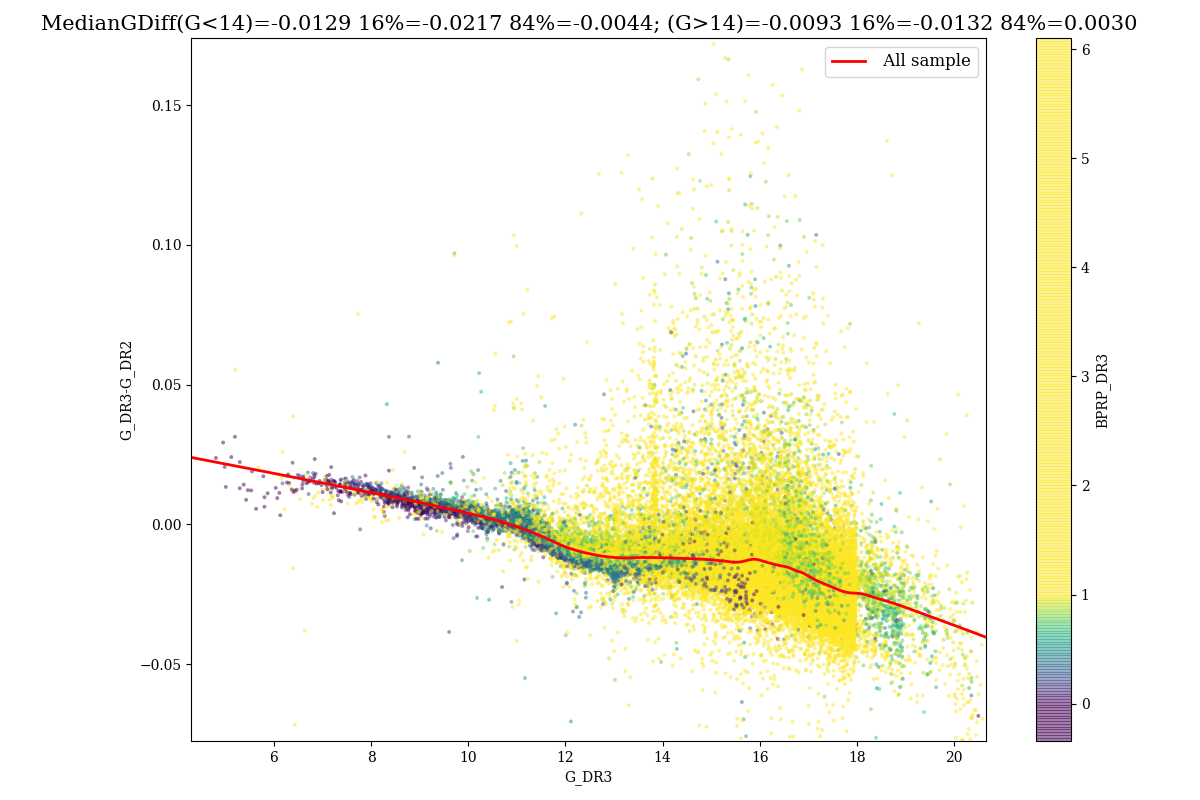Figure 14.77: Difference between GGaia⁢DR3-GGaia⁢DR2. The red line is the LOWESS of the data.

## Completeness in crowded areas

We derive the completeness for 26 globular clusters comparing Gaia DR3 data with HST completeness Sarajedini et al. (2007). The procedure and the sample are the same already used for Gaia DR2. We remember that the completeness of HST data is derived using crowding experiments and is higher than 90% in the whole Gaia range. Completeness table for all the clusters can be found in Fabricius et al. (2021). In Figure 14.78 we present the completeness as function of $G$.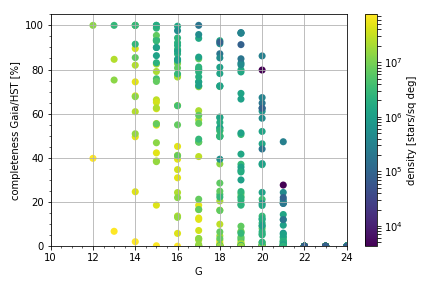Figure 14.78: Global Completeness as a function of G for the whole sample of globulars. The colour indicates the density.

A few significant cases are shown in Figures 14.79, 14.80, 14.81. In NGC 5053, the completeness in the inner and outer regions are very similar and quite high (60% at $G$ =20), in NGC 2298 the inner and outer regions have very different level of completeness. In the inner region, the completeness is about 10% at $G$ =20, and 60% in the outer region. In NGC 5286 the completeness in the inner region is 20% at $G$ $\sim$17.

It should be mentioned that the completeness of the astrometry only is generally higher. This is not an issue, but a feature of the data that depends on the processing. In globular clusters a percentage of about 20%-30% of stars have astrometry, but do not have $G_{\mathrm{BP}}-G_{\mathrm{RP}}$ colour. In NGC 6809 the percentage if of 37% (see Figure 14.82). Open clusters are more favourable cases. In general the percentage of stars missing $G_{\mathrm{BP}}-G_{\mathrm{RP}}$ is of the order of 1%-3% (see Figure 14.83). Some artefacts on the completeness showing the effect of the scanning law are visible (see Figure 14.83), but the number of cases is reduced in comparison to Gaia DR2.